# Electricity Class10 CBSE - MCQ test 2

Electricity Class10 CBSE - MCQ test 3
Q1. A battery of 10 volt carries 20,000 C of charge through a resistance of 20 Ω. The work done in 10 seconds is

2 × 10^3 joule

2 × 10^4 joule

2 × 10^5 joule

2 × 10^6 joule

3/5 ohm

5/3 ohm

5/6 ohm

6/5 ohm

Q3. To get 2 Ω resistance using only 6 Ω resistors, the number of them required is ....

2

3

5

None

Q4.Two device are connected between two points say A and B in parallel. The physical quantity that will remain the same between the two point is

Current

Voltage

Rasistance

None of these

Q5.Two device are connected between two points say A and B in series . The physical quantity that will remain the same between the two point is

Current

Voltage

Rasistence

All of the above

Q6. The least resistance obtained by using 2 Ω, 4 Ω, 1 Ω and 100 Ω is

< 100 Ω

< 4 Ω

< 1 Ω

> 2 Ω

Q7. Three resistances of 6, 10, and 20 ohms are connected in series. Find the equivalent resistance for the system.

36 ohm

32 ohm

18 ohm

None

Q8.The V-I graph of three resistors is shown below. Which resistor has maximum resistance?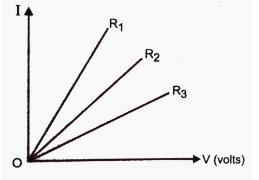R1

R2

R3

None of these

Q9. What is value the current flows through the 10 Ω resistor in the following circuit.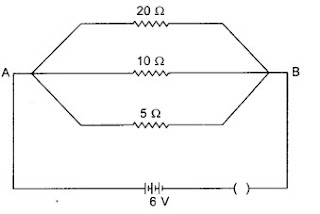1.2A

0.6A

0.2A

2A

Q10.What is measure of effective resistance between A and B is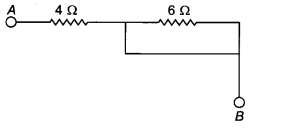4Ω

6Ω

10Ω

None of these

Q11. A cell, a resistor, a key, and an ammeter are arranged as shown in the circuit diagrams. The current recorded in the ammeter will be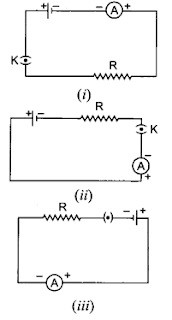maximum in (i)

maximum in (ii)

maximum in (iii)

same in all the cases

Q12.Which rasistance follow least current in the following circuit.20 Ω

10 Ω

5 Ω

None of these

Q13. What is the maximum resistance which can be made using five resistors each of 1/5 Ω?

1Ω

2Ω

3Ω

5Ω

Q14. The resistivity does not change if

the material is changed.

the temperature is changed

the shape of the resistor is changed.

both material and temperature are changed.

Q15. Electric potential is a:

scalar quantity

vector quantity

neither scalar nor vector

sometimes scalar and sometimes vector

Q16.1 mV is equal to:

10 volt

1000 volt

10^-3 volt

10^-6 volt

Q17. The unit of e.m.f. of a cell is

dyne

volt

ampere

joule

Q18. Conventionally, the direction of the current is taken as

the direction of flow of negative charge

the direction of flow of atoms

the direction of flow of molecules

the direction of flow of positive charge

Q19. The slope of voltage (V) versus current (I) is called -----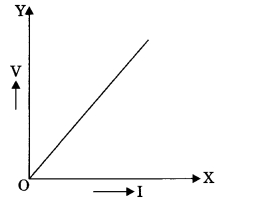voltage

rasistence

current

none of the above

Q20. The variable resistance is called..............

resistor

rheostat

open switch

none of these